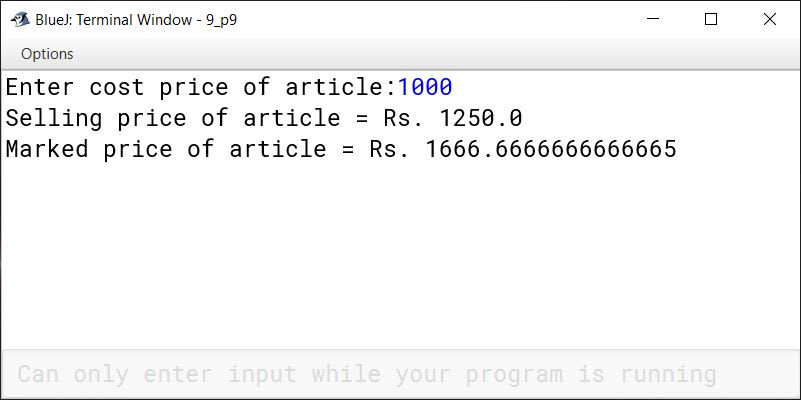# Input in Java

## Multiple Choice Questions

#### Question 1

Which of the following is the right way to use a comment in Java program?

1. /* comment */
2. /* comment
3. // comment //
4. */ comment */

/* comment */

Reason — /* comment */ is used to write multi line comments.

#### Question 2

Given: int p=55/0;
Name the type of error in the given statement:

1. Syntax
2. Logical
3. Runtime
4. Executional

Runtime

Reason — Division by 0 will result in an infinite value, which is a runtime error.

#### Question 3

The ............... method will accept a character using stream class.

Reason — (char)(in.read( )) method will accept a character using stream class.

#### Question 4

Which of the following method will accept a string by using scanner object?

1. next( )
3. next.String( )
4. next.char( )

next( )

Reason — next( ) method will accept a string by using scanner object.

#### Question 5

Which of the following is a method of Java Scanner class?

1. nextline( )
2. nextLine( )
3. Nextline( )
4. NextLine( )

nextLine( )

Reason — nextLine( ) is a method of Java Scanner class.

## Fill in the blanks

#### Question 1

The package needed to import StreamReader class is java.io.

#### Question 2

nextInt() function accepts an integer by using scanner object.

#### Question 3

The package needed to import Scanner class is java.util.

#### Question 4

The keyword import is used to include a package in a program.

#### Question 5

Assigning a value of 24.3 to a variable that is defined as int type is syntax error.

#### Question 6

input statement is used to accept value at run time.

#### Question 7

Scanner ob = new Scanner(System.in).

#### Question 8

The command line argument accepts the data value as an array of Strings.

## Explain the following functions

#### Question 1

This statement accepts an integer from the user using Stream class and parses it into int data type using Integer.parseInt() method.

#### Question 2

This statement reads a character using Stream class and casts it explicitly into char data type.

#### Question 3

next();

next() method accepts a string from the user as a word using Scanner class.

#### Question 4

public static void main(int b)

The given function accepts an integer value from the user at the time of execution of the program. The value for variable b must be provided as arguments to the main() function.

#### Question 5

nextLine();

nextLine() method accepts a string from the user as a line of text using Scanner class.

#### Question 1

What are the different ways to give input in a Java Program?

Java provides the following ways to give input in a program:

1. Using Function Argument
3. Using Scanner class
4. Using Command Line Arguments

#### Question 2

What is a package? Give an example.

In Java, a package is used to group related classes. Packages are of 2 types:

1. Built-In packages — These are provided by Java API
2. User-Defined packages — These are created by the programmers to efficiently structure their code.

For example, java.util, java.lang are built-in packages.

#### Question 3

What is the use of the keyword 'import' in Java programming?

'import' keyword is used to import built-in and user-defined packages into our Java programs.

#### Question 4

What is a Runtime error? Explain with an example.

Errors that occur during the execution of the program primarily due to the state of the program which can only be resolved at runtime are called Runtime errors.
Consider the below example:

```import java.util.Scanner;
class RunTimeError
{
public static void main(String args[]) {
Scanner in = new Scanner(System.in);
System.out.print("Enter a number: ");
int n = in.nextInt();
int result = 100 / n;
System.out.println("Result = " + result);
}
}```

This program will work fine for all non-zero values of n entered by the user. When the user enters zero, a run-time error will occur as the program is trying to perform an illegal mathematical operation of division by 0. When we are compiling the program, we cannot say if division by 0 error will occur or not. It entirely depends on the state of the program at run-time.

#### Question 5

What are the different types of errors that take place during the execution of a program? Name them.

Logical errors and Run-Time errors occur during the execution of the program.

#### Question 6

Give two differences between Syntax error and Logical error.

Syntax errorLogical error
Syntax errors occur when we violate the rules of writing the statements of the programming language.Logical errors occur due to our mistakes in programming logic.
Program fails to compile and execute.Program compiles and executes but doesn't give the desired output.
Syntax errors are caught by the compiler.Logical errors need to be found and corrected by people working on the program.

## Solutions to Unsolved Java Programs

#### Question 1

In an election, there are two candidates X and Y. On the election day, 80% of the voters go for polling, out of which 60% vote for X. Write a program to take the number of voters as input and calculate:

```import java.util.Scanner;

public class KboatPolling
{
public static void main(String args[]) {

Scanner in = new Scanner(System.in);

System.out.print("Enter the number of voters: ");
long totalVoters = in.nextLong();

long votedVoters = Math.round(0.8 * totalVoters);
long xVotes = Math.round(0.6 * votedVoters);

}
}```
##### Output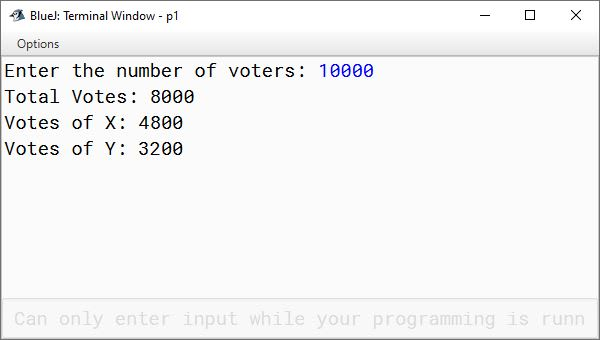#### Question 2

A shopkeeper offers 10% discount on the printed price of a mobile phone. However, a customer has to pay 9% GST on the remaining amount. Write a program in Java to calculate the amount to be paid by the customer taking printed price as an input.

```import java.util.Scanner;

public class KboatInvoice
{
public static void main(String args[]) {

Scanner in = new Scanner(System.in);

System.out.print("Enter the MRP: ");
double mrp = in.nextDouble();

double priceAfterDiscount = mrp - (0.1 * mrp);
double priceWithGST = priceAfterDiscount + (priceAfterDiscount * 0.09);

System.out.println("Price after 10% discount and 9% GST: " + priceWithGST);
}
}```
##### Output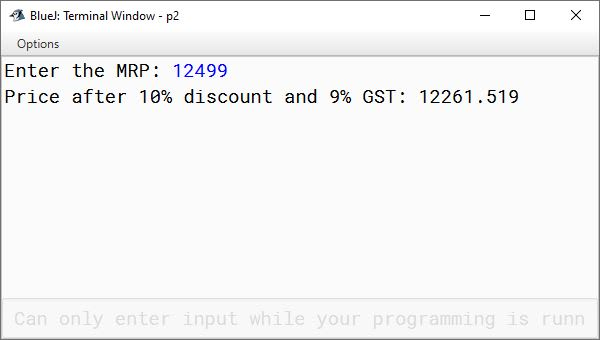#### Question 3

A man spends (1/2) of his salary on food, (1/15) on rent, (1/10) on miscellaneous activities. Rest of the salary is his saving. Write a program to calculate and display the following:

1. money spent on food
2. money spent on rent
3. money spent on miscellaneous activities
4. money saved

Take the salary as an input.

```import java.util.Scanner;

public class KboatSalary
{
public static void main(String args[]) {

Scanner in = new Scanner(System.in);

System.out.print("Enter the Salary: ");
float salary = in.nextFloat();

float foodSpend = salary / 2;
float rentSpend = salary / 15;
float miscSpend = salary / 10;
float savings = salary - (foodSpend + rentSpend + miscSpend);

System.out.println("Money spent on food: " + foodSpend);
System.out.println("Money spent on rent: " + rentSpend);
System.out.println("Money spent on miscellaneous: " + miscSpend);
System.out.println("Money saved: " + savings);
}
}```
##### Output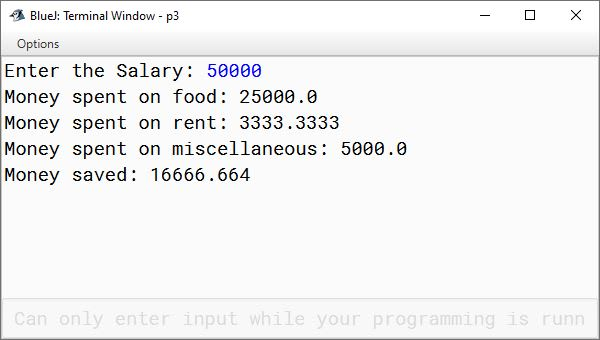#### Question 4

Write a program to input time in seconds. Display the time after converting them into hours, minutes and seconds.
Sample Input: Time in seconds: 5420
Sample Output: 1 Hour 30 Minutes 20 Seconds

```import java.util.Scanner;

public class KboatTime
{
public static void main(String args[]) {

Scanner in = new Scanner(System.in);

System.out.print("Time in seconds: ");
int inputTime = in.nextInt();

int hrs = inputTime / 3600;
int mins = (inputTime % 3600) / 60;
int secs = (inputTime % 3600) % 60;

System.out.println(hrs
+ " Hours "
+ mins
+ " Minutes "
+ secs
+ " Seconds");
}
}```
##### Output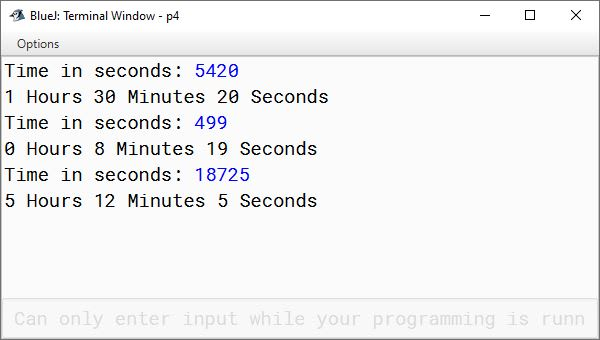#### Question 5

The driver took a drive to a town 240 km at a speed of 60 km/h. Later in the evening, he drove back at 20 km/h less than the usual speed. Write a program to calculate:

1. the total time taken by the driver
2. the average speed during the whole journey

[Hint: average speed = total distance / total time]

```public class KboatJourney
{
public static void main(String args[]) {

int distance = 240;
float speed = 60.0f;
float returnSpeed = speed - 20;

float time2Reach = distance / speed;
float time2Return = distance / returnSpeed;
float totalTime = time2Reach + time2Return;

float avgSpeed = (distance * 2) / totalTime;

System.out.println("Total time: " + totalTime);
System.out.println("Average speed: " + avgSpeed);
}
}```
##### Output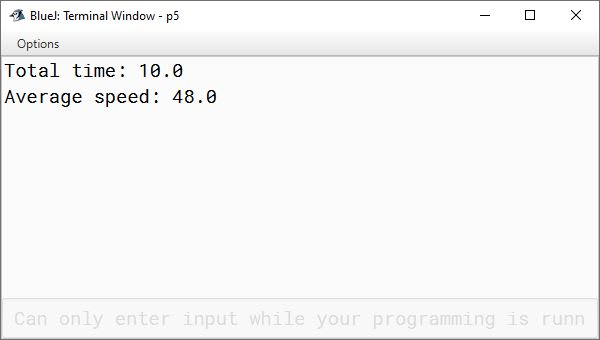#### Question 6

Write a program to input two unequal numbers. Display the numbers after swapping their values in the variables without using a third variable.
Sample Input: a = 76, b = 65
Sample Output: a = 65, b = 76

```import java.util.Scanner;

public class KboatNumberSwap
{
public static void main(String args[]) {

Scanner in = new Scanner(System.in);

System.out.print("Enter the first number: ");
int firstNum = in.nextInt();

System.out.print("Enter the second number: ");
int secondNum = in.nextInt();

if (firstNum == secondNum) {
System.out.println("Invalid Input. Numbers are equal.");
return;
}

firstNum = firstNum + secondNum;
secondNum = firstNum - secondNum;
firstNum = firstNum - secondNum;

System.out.println("First Number: " + firstNum);
System.out.println("Second Number: " + secondNum);
}
}```
##### Output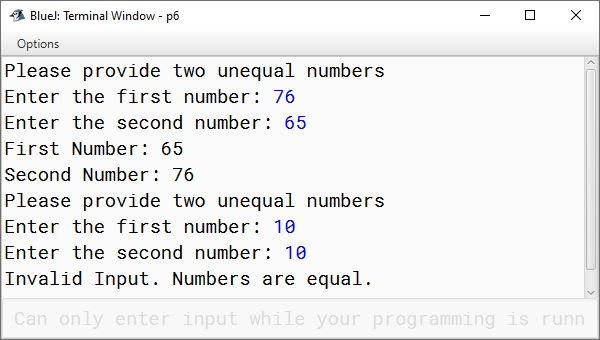#### Question 7

A certain amount of money is invested for 3 years at the rate of 6%, 8% and 10% per annum compounded annually. Write a program to calculate:

1. the amount after 3 years.
2. the compound interest after 3 years.

Accept certain amount of money (Principal) as an input.
Hint: A = P * (1 + (R1 / 100))T * (1 + (R2 / 100))T * (1 + (R3 / 100))T and CI = A - P

```import java.util.Scanner;

public class KboatCompoundInterest
{
public static void main(String args[]) {

Scanner in = new Scanner(System.in);

System.out.print("Enter the principal: ");
double principal = in.nextDouble();
System.out.println(principal);

float r1 = 6.0f, r2 = 8.0f, r3 = 10.0f;

double amount = principal * (1 + (r1 / 100)) * (1 + (r2 / 100)) * (1 + (r3 / 100));
double ci = amount - principal;

System.out.println("Amount after 3 years: " + amount);
System.out.println("Compound Interest: " + ci);
}
}```
##### Output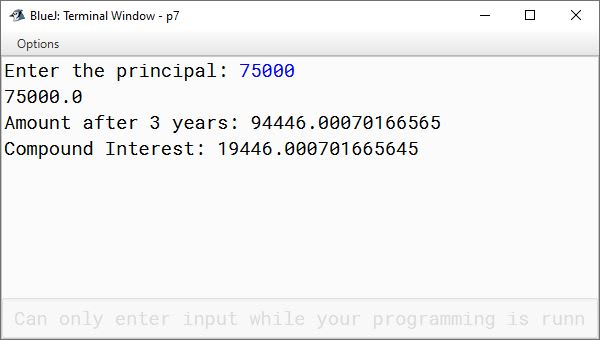#### Question 8

The co-ordinates of two points A and B on a straight line are given as (x1,y1) and (x2,y2). Write a program to calculate the slope (m) of the line by using formula:
Slope = (y2 - y1) / (x2 - x1)
Take the co-ordinates (x1,y1) and (x2,y2) as input.

```import java.util.Scanner;

public class KboatLineSlope
{
public static void main(String args[]) {

Scanner in = new Scanner(System.in);

System.out.print("Enter x coordinate of point A: ");
int x1 = in.nextInt();

System.out.print("Enter y coordinate of point A: ");
int y1 = in.nextInt();

System.out.print("Enter x coordinate of point B: ");
int x2 = in.nextInt();

System.out.print("Enter y coordinate of point B: ");
int y2 = in.nextInt();

float lineSlope = (y2 - y1) / (float)(x2 - x1);

System.out.println("Slope of line: " + lineSlope);
}
}```
##### Output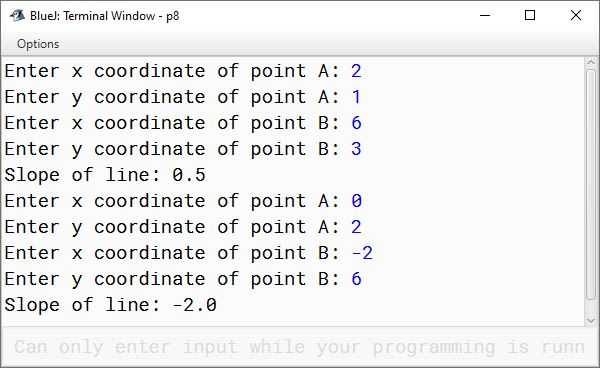#### Question 9

A dealer allows his customers a discount of 25% and still gains 25%. Write a program to input the cost of an article and display its selling price and marked price.

Hint: SP = ((100 + p%) / 100) * CP and MP = (100 / (100 - d%)) * SP

```import java.util.*;

public class KboatCalculatePrice
{
public static void main(String args[])
{
Scanner in = new Scanner(System.in);

System.out.print("Enter cost price of article:");
double cp = in.nextDouble();

int disc = 25, profit = 25;
double sp = 0.0, mp = 0.0;

sp = ((100 + profit) / 100.0) * cp;
System.out.println("Selling price of article = Rs. " + sp);

mp = (100.0 / (100 - disc)) * sp;
System.out.println("Marked price of article = Rs. " + mp);
}
}```
##### Output